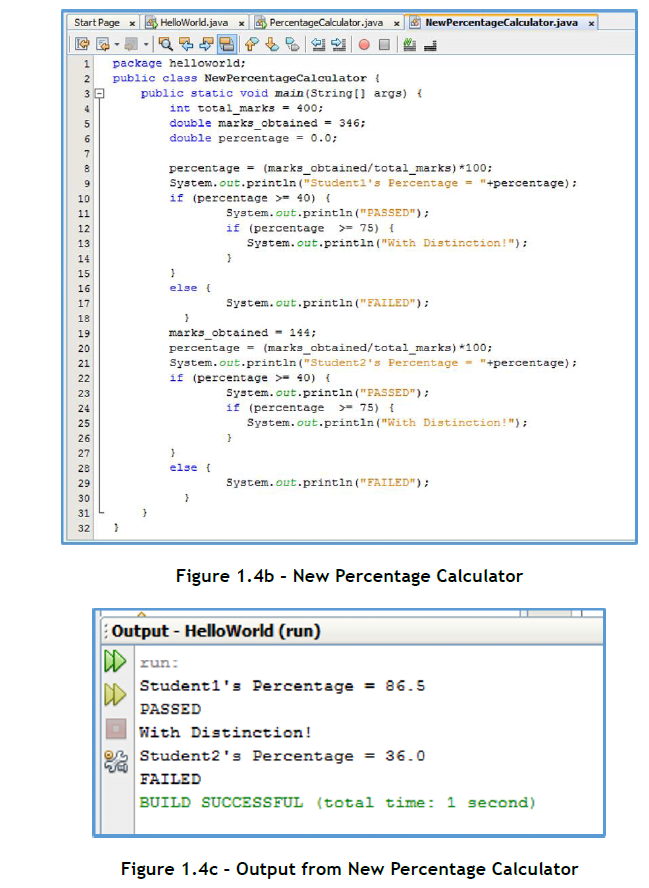Home » Online Computer Education » Learn Fundamentals of Java Programming “The if else Statement in Java Programming ” Lesson 9

# Learn Fundamentals of Java Programming “The if else Statement in Java Programming ” Lesson 9

The if else Statement

The if statement in Java lets us execute a block of code depending upon whether an expression evaluates to true or false. The structure of the Java if statement is as below:

if (expression) {
statements
}

The expression given in the round brackets after the if keyword, is a condition – an expression constructed using relational and logical operators. If the condition evaluates to true, the block of code following the if is executed, otherwise not. If there is only one statement in the block after the if , the curly braces are optional. Let us enhance our percentage calculator program. If the percentage secured by the student is more than or equal to 40%, we will declare the student as passed. So, in our percentage calculator program, we write,

if (percentage > = 40) {
System.out.println(“PASSED “);
}

If we also want to display “FAILED” when the student has scored less than 40%, we use the if else statement. The structure of the Java if else statement is as below:

If (expression) {
statements
}
else {
statements
}

As before, the block of code following the if is executed if the conditional expression evaluates to true. The block of code following the else is executed if the conditional expression evaluates to false. In our percentage calculator program, we can write,

if (percentage > = 40) {
System.out.println(“PASSED “);
}
else {
System.out.println(“FAIL ED “);
}

Sometimes, you may want to execute a block of code based on the evaluation of two or more conditional expressions. For this, you can combine expressions using the logical && or || operators. Let’s say, we want to print the division with which the student has passed, then, we can write the following code. The first if statement uses the logical AND (&&) operator to combine two relational expressions.

if ((percentage > = 40) && (percentage < 60)) {
System.out.println(“PASSED with II Division “);
}
if (percentage > = 6 0) {
System.out.println(“PASSED with I Division “);
}
if (percentage < 40) {
System.out.println(“FAILED “);
}

If-else statements can also be nested – you can have another if-else statement within an outer if or else statement. The example below is a nested if.

if (percentage > = 40) {
System.out.println(“PASSED “);
if (percentage >= 75) {
System.out.println(“With Distinction !”);
}
}
else {
System.out.println(“FAILED “);
}

You can see the complete code listing of the new Percentage Calculator program in Figure 1.2g and its output in Figure 1.2h.The main objective of this website is to provide quality study material to all students (from 1st to 12th class of any board) irrespective of their background as our motto is “Education for Everyone”. It is also a very good platform for teachers who want to share their valuable knowledge.